# 1.2 Domain and range  (Page 8/11)

 Page 8 / 11

## Verbal

Why does the domain differ for different functions?

The domain of a function depends upon what values of the independent variable make the function undefined or imaginary.

How do we determine the domain of a function defined by an equation?

Explain why the domain of $\text{\hspace{0.17em}}f\left(x\right)=\sqrt{x}\text{\hspace{0.17em}}$ is different from the domain of $\text{\hspace{0.17em}}f\left(x\right)=\sqrt[]{x}.$

There is no restriction on $\text{\hspace{0.17em}}x\text{\hspace{0.17em}}$ for $\text{\hspace{0.17em}}f\left(x\right)=\sqrt{x}\text{\hspace{0.17em}}$ because you can take the cube root of any real number. So the domain is all real numbers, $\text{\hspace{0.17em}}\left(-\infty ,\infty \right).\text{\hspace{0.17em}}$ When dealing with the set of real numbers, you cannot take the square root of negative numbers. So $\text{\hspace{0.17em}}x$ -values are restricted for $\text{\hspace{0.17em}}f\left(x\right)=\sqrt[]{x}\text{\hspace{0.17em}}$ to nonnegative numbers and the domain is $\text{\hspace{0.17em}}\left[0,\infty \right).$

When describing sets of numbers using interval notation, when do you use a parenthesis and when do you use a bracket?

How do you graph a piecewise function?

Graph each formula of the piecewise function over its corresponding domain. Use the same scale for the $\text{\hspace{0.17em}}x$ -axis and $\text{\hspace{0.17em}}y$ -axis for each graph. Indicate inclusive endpoints with a solid circle and exclusive endpoints with an open circle. Use an arrow to indicate $\text{\hspace{0.17em}}-\infty \text{\hspace{0.17em}}$ or Combine the graphs to find the graph of the piecewise function.

## Algebraic

For the following exercises, find the domain of each function using interval notation.

$f\left(x\right)=-2x\left(x-1\right)\left(x-2\right)$

$f\left(x\right)=5-2{x}^{2}$

$\left(-\infty ,\infty \right)$

$f\left(x\right)=3\sqrt{x-2}$

$f\left(x\right)=3-\sqrt{6-2x}$

$\left(-\infty ,3\right]$

$f\left(x\right)=\sqrt{4-3x}$

$\begin{array}{l}\\ f\left(x\right)=\sqrt[]{{x}^{2}+4}\end{array}$

$\left(-\infty ,\infty \right)$

$f\left(x\right)=\sqrt{1-2x}$

$f\left(x\right)=\sqrt{x-1}$

$\left(-\infty ,\infty \right)$

$f\left(x\right)=\frac{9}{x-6}$

$f\left(x\right)=\frac{3x+1}{4x+2}$

$\left(-\infty ,-\frac{1}{2}\right)\cup \left(-\frac{1}{2},\infty \right)$

$f\left(x\right)=\frac{\sqrt{x+4}}{x-4}$

$f\left(x\right)=\frac{x-3}{{x}^{2}+9x-22}$

$\left(-\infty ,-11\right)\cup \left(-11,2\right)\cup \left(2,\infty \right)$

$f\left(x\right)=\frac{1}{{x}^{2}-x-6}$

$f\left(x\right)=\frac{2{x}^{3}-250}{{x}^{2}-2x-15}$

$\left(-\infty ,-3\right)\cup \left(-3,5\right)\cup \left(5,\infty \right)$

$\frac{5}{\sqrt{x-3}}$

$\frac{2x+1}{\sqrt{5-x}}$

$\left(-\infty ,5\right)$

$f\left(x\right)=\frac{\sqrt{x-4}}{\sqrt{x-6}}$

$f\left(x\right)=\frac{\sqrt{x-6}}{\sqrt{x-4}}$

$\left[6,\infty \right)$

$f\left(x\right)=\frac{x}{x}$

$f\left(x\right)=\frac{{x}^{2}-9x}{{x}^{2}-81}$

$\left(-\infty ,-9\right)\cup \left(-9,9\right)\cup \left(9,\infty \right)$

Find the domain of the function $\text{\hspace{0.17em}}f\left(x\right)=\sqrt{2{x}^{3}-50x}\text{\hspace{0.17em}}$ by:

1. using algebra.
2. graphing the function in the radicand and determining intervals on the x -axis for which the radicand is nonnegative.

## Graphical

For the following exercises, write the domain and range of each function using interval notation.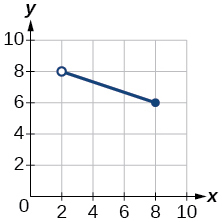domain: $\text{\hspace{0.17em}}\left(2,8\right],\text{\hspace{0.17em}}$ range $\text{\hspace{0.17em}}\left[6,8\right)\text{\hspace{0.17em}}$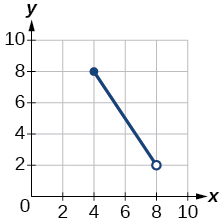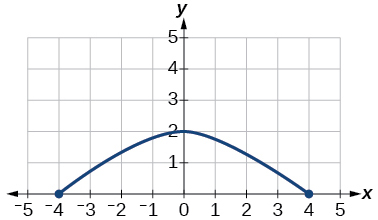domain: range: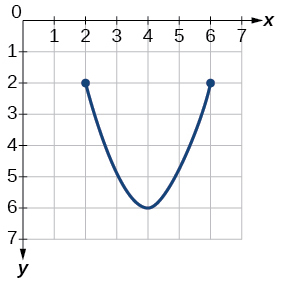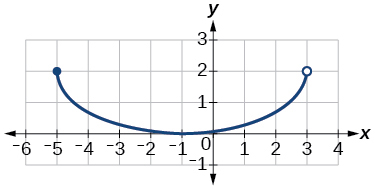domain: range: $\text{\hspace{0.17em}}\left[0,2\right]$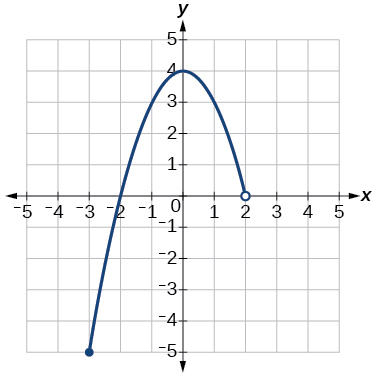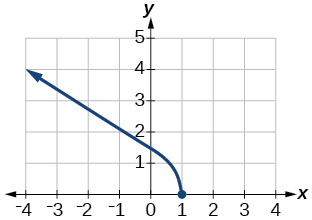domain: $\text{\hspace{0.17em}}\left(-\infty ,1\right],\text{\hspace{0.17em}}$ range: $\text{\hspace{0.17em}}\left[0,\infty \right)\text{\hspace{0.17em}}$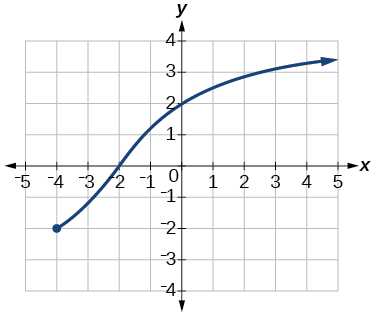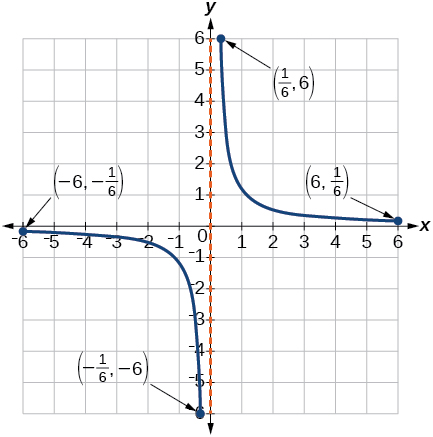domain: $\text{\hspace{0.17em}}\left[-6,-\frac{1}{6}\right]\cup \left[\frac{1}{6},6\right];\text{\hspace{0.17em}}$ range: $\text{\hspace{0.17em}}\left[-6,-\frac{1}{6}\right]\cup \left[\frac{1}{6},6\right]\text{\hspace{0.17em}}$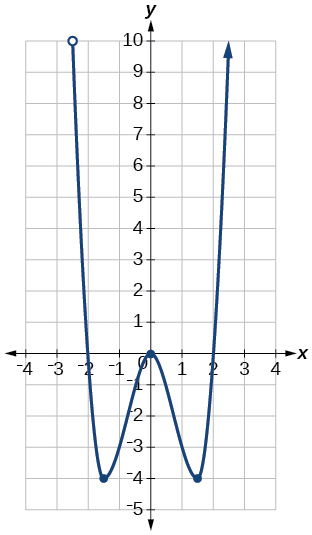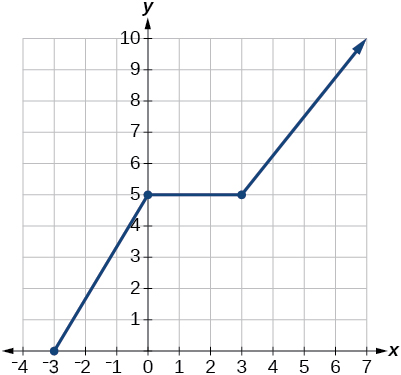domain: range: $\text{\hspace{0.17em}}\left[0,\infty \right)\text{\hspace{0.17em}}$

For the following exercises, sketch a graph of the piecewise function. Write the domain in interval notation.

$f\left(x\right)=\left\{\begin{array}{lll}x+1\hfill & \text{if}\hfill & x<-2\hfill \\ -2x-3\hfill & \text{if}\hfill & x\ge -2\hfill \end{array}$

$f\left(x\right)=\left\{\begin{array}{lll}2x-1\hfill & \text{if}\hfill & x<1\hfill \\ 1+x\hfill & \text{if}\hfill & x\ge 1\hfill \end{array}$

domain: $\text{\hspace{0.17em}}\left(-\infty ,\infty \right)$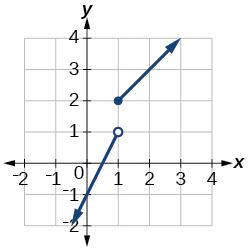$f\left(x\right)=\left\{\begin{array}{c}x+1\text{\hspace{0.17em}}\text{\hspace{0.17em}}\text{if}\text{\hspace{0.17em}}\text{\hspace{0.17em}}x<0\\ x-1\text{\hspace{0.17em}}\text{\hspace{0.17em}}\text{if}\text{\hspace{0.17em}}\text{\hspace{0.17em}}\text{\hspace{0.17em}}x>0\end{array}$

$f\left(x\right)=\left\{\begin{array}{ccc}3& \text{if}& x<0\\ \sqrt{x}& \text{if}& x\ge 0\end{array}$

domain: $\text{\hspace{0.17em}}\left(-\infty ,\infty \right)$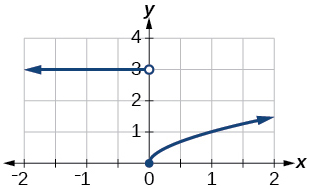$f\left(x\right)=\left\{\begin{array}{r}\hfill \begin{array}{r}\hfill {x}^{2}\\ \hfill x+2\end{array}\end{array}\text{\hspace{0.17em}}\text{\hspace{0.17em}}\begin{array}{l}\text{if}\text{\hspace{0.17em}}\text{\hspace{0.17em}}\text{\hspace{0.17em}}\text{\hspace{0.17em}}\text{\hspace{0.17em}}x<0\hfill \\ \text{if}\text{\hspace{0.17em}}\text{\hspace{0.17em}}\text{\hspace{0.17em}}\text{\hspace{0.17em}}\text{\hspace{0.17em}}x\ge 0\hfill \end{array}$

domain: $\text{\hspace{0.17em}}\left(-\infty ,\infty \right)$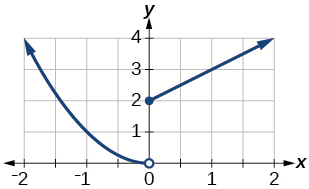$f\left(x\right)=\left\{\begin{array}{ccc}x+1& \text{if}& x<1\\ {x}^{3}& \text{if}& x\ge 1\end{array}$

$f\left(x\right)=\left\{\begin{array}{c}|x|\\ 1\end{array}\begin{array}{l}\text{\hspace{0.17em}}\text{\hspace{0.17em}}\text{\hspace{0.17em}}\text{if}\text{\hspace{0.17em}}\text{\hspace{0.17em}}\text{\hspace{0.17em}}x<2\hfill \\ \text{\hspace{0.17em}}\text{\hspace{0.17em}}\text{\hspace{0.17em}}\text{if}\text{\hspace{0.17em}}\text{\hspace{0.17em}}\text{\hspace{0.17em}}x\ge 2\hfill \end{array}$

domain: $\text{\hspace{0.17em}}\left(-\infty ,\infty \right)$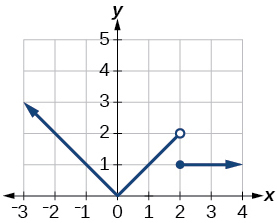## Numeric

For the following exercises, given each function $f,$ evaluate $f\left(-3\right),\text{\hspace{0.17em}}f\left(-2\right),\text{\hspace{0.17em}}f\left(-1\right),$ and $f\left(0\right).$

$f\left(x\right)=\left\{\begin{array}{lll}x+1\hfill & \text{if}\hfill & x<-2\hfill \\ -2x-3\hfill & \text{if}\hfill & x\ge -2\hfill \end{array}$

$\begin{array}{cccc}f\left(-3\right)=1;& f\left(-2\right)=0;& f\left(-1\right)=0;& f\left(0\right)=0\end{array}$

For the following exercises, given each function $\text{\hspace{0.17em}}f,\text{\hspace{0.17em}}$ evaluate $f\left(-1\right),\text{\hspace{0.17em}}f\left(0\right),\text{\hspace{0.17em}}f\left(2\right),\text{\hspace{0.17em}}$ and $\text{\hspace{0.17em}}f\left(4\right).$

$f\left(x\right)=\left\{\begin{array}{lll}7x+3\hfill & \text{if}\hfill & x<0\hfill \\ 7x+6\hfill & \text{if}\hfill & x\ge 0\hfill \end{array}$

$\begin{array}{cccc}f\left(-1\right)=-4;& f\left(0\right)=6;& f\left(2\right)=20;& f\left(4\right)=34\end{array}$

$f\left(x\right)=\left\{\begin{array}{ccc}{x}^{2}-2& \text{if}& x<2\\ 4+|x-5|& \text{if}& x\ge 2\end{array}$

$f\left(x\right)=\left\{\begin{array}{ccc}5x& \text{if}& x<0\\ 3& \text{if}& 0\le x\le 3\\ {x}^{2}& \text{if}& x>3\end{array}$

$\begin{array}{cccc}f\left(-1\right)=-5;& f\left(0\right)=3;& f\left(2\right)=3;& f\left(4\right)=16\end{array}$

For the following exercises, write the domain for the piecewise function in interval notation.

domain: $\text{\hspace{0.17em}}\left(-\infty ,1\right)\cup \left(1,\infty \right)$

$f\left(x\right)=\left\{\begin{array}{c}2x-3\\ -3{x}^{2}\end{array}\text{\hspace{0.17em}}\text{\hspace{0.17em}}\begin{array}{c}\text{if}\text{\hspace{0.17em}}\text{\hspace{0.17em}}\text{\hspace{0.17em}}x<0\\ \text{if}\text{\hspace{0.17em}}\text{\hspace{0.17em}}\text{\hspace{0.17em}}x\ge 2\end{array}$

## Technology

Graph $\text{\hspace{0.17em}}y=\frac{1}{{x}^{2}}\text{\hspace{0.17em}}$ on the viewing window $\text{\hspace{0.17em}}\left[-0.5,-0.1\right]\text{\hspace{0.17em}}$ and $\text{\hspace{0.17em}}\left[0.1,0.5\right].\text{\hspace{0.17em}}$ Determine the corresponding range for the viewing window. Show the graphs.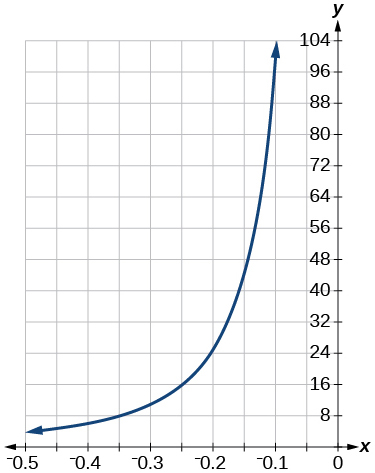window: $\text{\hspace{0.17em}}\left[-0.5,-0.1\right];\text{\hspace{0.17em}}$ range: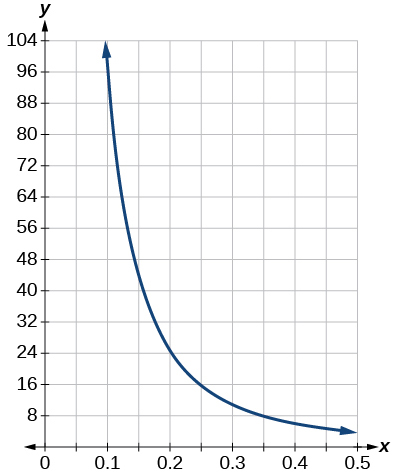window: range:

Graph $\text{\hspace{0.17em}}y=\frac{1}{x}\text{\hspace{0.17em}}$ on the viewing window $\text{\hspace{0.17em}}\left[-0.5,-0.1\right]\text{\hspace{0.17em}}$ and Determine the corresponding range for the viewing window. Show the graphs.

## Extension

Suppose the range of a function $\text{\hspace{0.17em}}f\text{\hspace{0.17em}}$ is What is the range of $\text{\hspace{0.17em}}|f\left(x\right)|?$

Create a function in which the range is all nonnegative real numbers.

Create a function in which the domain is $\text{\hspace{0.17em}}x>2.$

Many answers. One function is $\text{\hspace{0.17em}}f\left(x\right)=\frac{1}{\sqrt{x-2}}.$

## Real-world applications

The height $\text{\hspace{0.17em}}h\text{\hspace{0.17em}}$ of a projectile is a function of the time $\text{\hspace{0.17em}}t\text{\hspace{0.17em}}$ it is in the air. The height in feet for $\text{\hspace{0.17em}}t\text{\hspace{0.17em}}$ seconds is given by the function $h\left(t\right)=-16{t}^{2}+96t.$ What is the domain of the function? What does the domain mean in the context of the problem?

The domain is it takes 6 seconds for the projectile to leave the ground and return to the ground

The cost in dollars of making $\text{\hspace{0.17em}}x\text{\hspace{0.17em}}$ items is given by the function $\text{\hspace{0.17em}}C\left(x\right)=10x+500.$

1. The fixed cost is determined when zero items are produced. Find the fixed cost for this item.
2. What is the cost of making 25 items?
3. Suppose the maximum cost allowed is \$1500. What are the domain and range of the cost function, $\text{\hspace{0.17em}}C\left(x\right)?$

how fast can i understand functions without much difficulty
what is set?
a colony of bacteria is growing exponentially doubling in size every 100 minutes. how much minutes will it take for the colony of bacteria to triple in size
I got 300 minutes. is it right?
Patience
no. should be about 150 minutes.
Jason
It should be 158.5 minutes.
Mr
ok, thanks
Patience
100•3=300 300=50•2^x 6=2^x x=log_2(6) =2.5849625 so, 300=50•2^2.5849625 and, so, the # of bacteria will double every (100•2.5849625) = 258.49625 minutes
Thomas
what is the importance knowing the graph of circular functions?
can get some help basic precalculus
What do you need help with?
Andrew
how to convert general to standard form with not perfect trinomial
can get some help inverse function
ismail
Rectangle coordinate
how to find for x
it depends on the equation
Robert
yeah, it does. why do we attempt to gain all of them one side or the other?
Melissa
whats a domain
The domain of a function is the set of all input on which the function is defined. For example all real numbers are the Domain of any Polynomial function.
Spiro
Spiro; thanks for putting it out there like that, 😁
Melissa
foci (–7,–17) and (–7,17), the absolute value of the differenceof the distances of any point from the foci is 24.
difference between calculus and pre calculus?
give me an example of a problem so that I can practice answering
x³+y³+z³=42
Robert
dont forget the cube in each variable ;)
Robert
of she solves that, well ... then she has a lot of computational force under her command ....
Walter
what is a function?
I want to learn about the law of exponent
explain this

#### Get Jobilize Job Search Mobile App in your pocket Now!ByByBy OpenStaxBy OpenStaxBy Robert MorrisBy OpenStaxBy David CoreyByBy Edgar DelgadoBy OpenStaxBy Brooke DelaneyBy Edgar Delgado